The hearing aid market is inelastic. This means that changes in price will have little impact relative to the number of potential hearing aid users who enter your practice. These inelastic forces, however, play an important role in the pricing strategy employed and its impact on revenues. Presented in this paper are price elasticity—and its impact on total revenue—for a hypothetical dispensing practice. Potential strategies to offset inelasticity in the clinical setting are also discussed.Amyn M. Amlani, PhD, is assistant professor of audiology and the director of the audiology undergraduate program at the Department of Speech & Hearing Sciences, University of North Texas, Denton.

The pricing structure within the hearing aid market is inelastic.1-3 This means that increasing or decreasing the retail price of hearing aids will have little effect on the end number of users.

Amlani and De Silva2 found that the demand function for hearing aids yielded a coefficient of -.49. In other words, as the price of hearing aids decreases by 10%, all else being equal, demand increases only by 4.9%. Similarly, Aaron1 found that decreasing the cost of hearing aids through government subsidies would yield an increase in demand of only -.35. That is, a 10% decrease in the price of hearing aids increases market demand only by 3.5%, all else being equal.

The difference in coefficients between these two studies stems largely from changes that have occurred in the marketplace over time (eg, miniaturization of style, incorporation of digital signal processing). In other words, as product differentiation increases, the market becomes more elastic (ie, a coefficient value closer to 1.0).

### Implications for Industry

The inelastic finding is also applicable to market penetration. In 2004, market penetration—the estimated number of hearing aid users (7.4 million) divided by the estimated number of persons with hearing loss (31.5 million)—was 23.5%.4 By applying the absolute value of the coefficient (49%) from Amlani and De Silva2 to the present market penetration of hearing aids (ie, 23.5%), the number of persons with hearing loss procuring devices would increase by only 11.5% (23.5% x 49%), resulting in a total market penetration of 35.0%. That is, the number of hearing aid users would increase from the estimated 7.4 million to a maximum of 11.0 million in today’s market. Stated differently, if hearing aids were given away by the government at no cost to the user, then 65% of the hearing-impaired population would decline the offer.

These results further imply that lowering the price of devices—a common business practice—will not result in appreciable increases in the number of devices dispensed, and increasing prices—often deemed immoral given the high retail price of these devices—will not decrease markedly the number of potential hearing aid users. This is contrary to the practice of many dispensing audiologists and otolaryngologists, who believe that simply reducing hearing aid prices will attract a larger number of potential users—particularly for lower-priced devices—ultimately increasing their total revenue. (Author’s note: Because expenses incurred by each practice are somewhat heterogeneous and because of differences in how retail prices of hearing aids are decided, this article uses the term total revenue. Total, or gross, revenue is determined by the retail price of a product multiplied by the quantity sold [Revenue = Price x Quantity Sold].)

Given the present demand function within the market, Amlani and De Silva2—using data from Kochkin5—found that the price of hearing aids should be increased at low prices because of their inelastic demand, and prices of more expensive devices should be decreased because of their elastic demand.

### Implications for Dispensers

The inelastic forces in the hearing aid market play an important role in the pricing strategy because revenue dictates the direction of a practice. Establishing a pricing strategy for hearing aids goes beyond bundled costs that typically cover expenses, such as the wholesale cost of each device, any overhead costs, and determining the appropriate fee for the dispensing professional’s time during hearing aid adjustment and rehabilitation. An effective pricing strategy should indicate, at a minimum, how potential users react toward a product (as measured by quantity demand) as a function of change in price.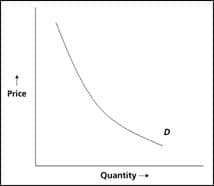FIGURE 1. The relationship between price and quantity for a hypothetical demand function (D).

This is known as price elasticity, which is a component of economic theory. By knowing at which price consumers are more (or less) sensitive, dispensers are better able to maximize their total revenue. While it is beyond the scope of this paper to provide a discussion on how to price hearing aids using either a cost- or marketing-based approach, the reader should be aware that pricing is the point at which marketing theory and economic theory converge. The purpose of this paper is to introduce the reader to the concept of price elasticity as it relates to the demand curve for a hypothetical practice and show the relationship between the demand curve and total revenue. Potential strategies to reduce the inelasticity in the clinical setting are also discussed.

### Price Elasticity of Demand

The law of demand is part of economic theory that presumes an inverse relationship between the amount of a product (or service) that consumers plan to purchase during a given time period at a particular price. This generalized relationship is illustrated in Figure 1. Note that, as quantity increases, price decreases along the demand function (D). Conversely, as quantity decreases, price increases along the same curve.

In addition to price, demand for a product increases or decreases due to external factors. These factors, and their impact on the hearing aid market, can be found in Amlani.6

As the relationship between quantity and price changes between two points, the slope of the demand function also changes. Calculating the percentage of change in the slope of the demand function provides information about the price elasticity of demand (e). In other words, e represents the sensitivity of consumer purchasing behavior when price is increased (or decreased) by some percentage and the corresponding percentage decrease (or increase) in quantity demanded.

e is calculated using the formula: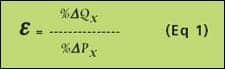where e is the price elasticity of demand, %ΔQx is the percentage change in the quantity demand of a product (or service) at point x, and %ΔPx is the percentage change in price at the same point. When e > |1|, the demand curve is elastic, suggesting that consumers are responsive to changes in price. On the other hand, when e < |1|, the demand curve is inelastic, indicating that consumers are not responsive to changes in price.

Equation 2 shows the calculation for %ΔQx: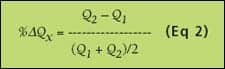where Q2 – Q1 yields the difference between quantities at two points, and (Q1 + Q2)/2 is the average quantity between the two quantities.

Similarly, %ΔPx is expressed as: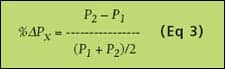where P2 – P1 yields the difference between prices at two points, and (P1 + P2)/2 is the average price between the two prices.

Lastly, the equation for calculating total revenue is:where R represents total revenue, P denotes price, and Q signifies quantity demanded. From Equation 4, the astute reader will note that changes in price, quantity demanded, or both, impact total revenue.

Total revenue is also impacted by e. Specifically, decreasing price in an elastic demand function will result in an increase in total revenue. Total revenue increases when the percentage change in quantity demand exceeds the percentage change in price. Alternatively, increased price in an inelastic demand function will result in an increase in total revenue. Total revenue decreases when the percentage change in price is greater than the percentage change in quantity demanded.

### Applying Economic Theory to Clinical Practice

Table 1 (page 16) shows data obtained from a hypothetical practice that dispensed 117 hearing aids during the previous fiscal year. The total revenue for this practice during this period was \$221,000.

Assume that this hypothetical practice is located in a competitive market, and in order to stay competitive, the practitioner has recently renovated the office and upgraded their equipment. To offset these new expenses, the practitioner projects that total revenue must be increased by \$15,000 over the next fiscal year. The previous year’s fiscal data, reported in Table 1, indicates that the majority of hearing aids (76%, 89/117) dispensed were less than \$2,500 per device. Prior to changing the price of hearing aids, the practitioner begins by determining the price point consumers are sensitive to relative to the retail cost of hearing aids. In other words, at which point can prices be increased (or decreased)?

The practitioner begins by assessing the price elasticity between the 26 units (Q1) sold at \$500 (P1) and the 20 units (Q2) sold at \$1,000 (P2). To calculate %ΔQ—from Equation 2—the numerator yields a value of -6 units (20 – 26) and the denominator equals 23 units ([26 + 20]/2). This yields a %ΔQ of -0.261. Next, %ΔP is calculated between the same two points, using Equation 3. The numerator yields \$500 (\$1,000 – \$500) and the denominator equals \$750 ([\$1,000 + \$500]/2), or a %ΔP of 0.667. Using Equation 1, e between these two points equals -0.391 (-0.261/0.667), which is inelastic. This means that consumers are not responsive to changes in price between these price points. Therefore, this practice could decrease or increase the price of hearing aids between these two price points with little or no change in consumer behavior.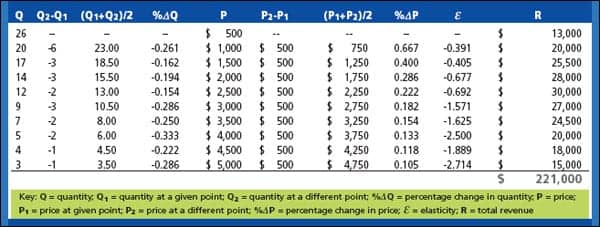TABLE 1. Data from a hypothetical practice for the previous fiscal year. See text for details.

Table 1 lists the various values and calculated results between the remaining points. Note that consumer behavior is inelastic for hearing aids priced up to \$2,500, and becomes elastic for prices at \$3,000 per unit and higher. This shift in consumer behavior is also depicted in the total revenue column of Table 1. Specifically, note that total revenue increases as price increases in the inelastic portion of the demand curve for prices ranging from \$500 per unit to \$2,500 per unit, and total revenue decreases for prices ranging between \$3,000 per unit and \$5,000 per unit in the elastic portion of the demand curve.

Given this information, this practice should increase the price of hearing aids in the inelastic portion of the demand curve with the expectation of a slight reduction in the number of patients who purchase hearing aids at these prices. Conversely, the practice should decrease the price of hearing aids in the elastic portion of the demand curve with the anticipation of a small increase in the number of patients who purchase higher-priced devices.TABLE 2. Forecasted data from the same hypothetical practice for the upcoming fiscal year. See text for details.

Table 2 is an estimated projection of quantity demanded as a function of change in price based on the findings shown in Table 1. Specifically, the practitioner estimates that a \$150 increase in price is more than sufficient to cover the \$15,000 expense assuming that the same quantity of devices will be dispensed (ie, 117). By increasing price, the practitioner projects that quantity demanded will decrease by two patients at each inelastic price point and increase by two patients at each elastic price point.

For both Tables 1 and 2, the total number of units dispensed remains the same (ie, 117). Total revenue in the upcoming fiscal year is estimated to increase by \$31,150 to \$252,150, up from \$221,000. The \$31,150 increase is slightly greater than double what is needed to cover the cost of the new expenses. Further, the number of lower-priced hearing aids is projected to be reduced by 10 units from 89 (76%) to 79 (68%). That is, an 8% decrease in the sale of lower-end hearing aids is expected to result in an 8.8% increase in total revenue.

### Summary

In this paper, the price elasticity of demand e was introduced so that practitioners could quantify the sensitivity of consumer purchasing behavior as it applies to hearing aids. Given the inelastic nature of the hearing aid market, the author hopes that the information provided in this article will help hearing health professionals to structure the price of hearing aids that maximizes the potential of their business.

### References

1. Aaron MJ. An Economic Study of the United States Hearing Aid Industry: A Demand- and Supply-Side Examination [unpublished doctoral dissertation]. Chicago: University of Illinois; 1987.
2. Amlani AM, De Silva DG. Effects of business cycles and FDA intervention on the hearing aid industry. Am J Audiol. 2005;14(1):71-79.
3. Lee K, Lotz P. Noise and silence in the hearing instrument industry. Working Paper, Department of Industrial Economics & Strategy, Copenhagen Business School; 1998.
4. Kochkin S. MarkeTrak VII: Hearing loss population tops 31 million people. Hearing Review. 2005;12(7):16-29.
5. Kochkin S. MarkeTrak III-Part 3: Would lower retail prices grow the market? Hear Jour. 1992;45(9):36-38.
6. Amlani AM. How patient demand impacts pricing and revenue. Hearing Review. 2008;15(3):16,18-19.

Correspondence can be addressed to or Amyn M. Amlani, PhD, at .